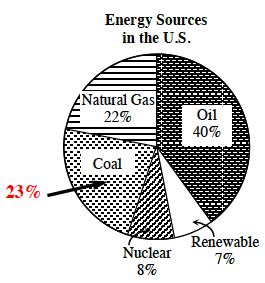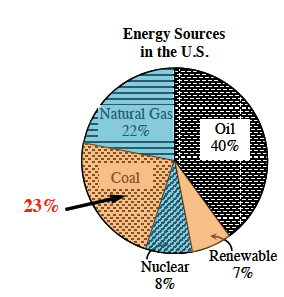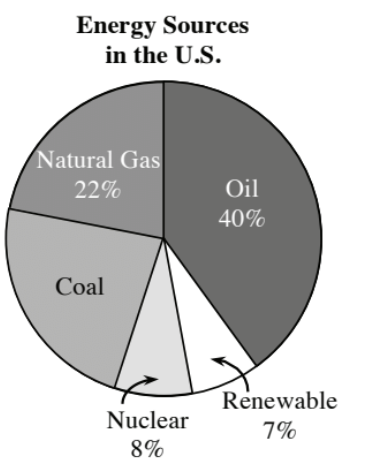### Home > CC3 > Chapter 7 > Lesson 7.1.1 > Problem7-7

7-7.

1. According to the graph, what percent of the energy in the United States comes from coal?

What percentage of energy remains out of $100\%$ if the other energy sources total to $77\%$?

$23\%$1. Which two sources of energy equal about one third of the total energy for the United States?

If one third is equal to about $30\%$, which two energy sources could add up to about this percentage for the total energy in the United States?1. What combination of energy sources provides about half of the total energy for the United States?

If half is equal to $50\%$, which energy sources could add up to around this percentage for the total energy in the United States?

Oil ($40\%$) and Nuclear ($8\%$) total to $48\%$. Renewable ($7\%$), Coal ($23\%$), and Natural Gas ($22\%$) total to $52\%$.